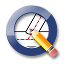QCAD Open Source 2D CAD
Math Module

This module contains fundamental classes that implement basic mathematical concepts such as vectors or matrices as well as basic geometrical classes that represent points, lines, triangles and other basic geometrical shapes. More...

Classes

class  RArc
Low-level mathematical representation of an arc. More...

class  RBox
Represents a box e.g. More...

class  RCircle
Low-level mathematical representation of a circle. More...

class  RDirected
Copyright (c) 2011-2018 by Andrew Mustun. More...

class  REllipse
Low-level mathematical representation of an ellipse or ellipse arc. More...

class  REllipseProxy
Proxy for advanced ellipse functionality. More...

class  RExplodable
Interface for explodable shape classes. More...

class  RLine
Low-level mathematical representation of a line. More...

class  RMath
Math functions. More...

class  RMatrix
Simple matrix class. More...

class  RPoint
Copyright (c) 2011-2018 by Andrew Mustun. More...

class  RPolyline
Low-level mathematical representation of an open polyline or closed polyline (= polygon). More...

class  RPolylineProxy
Proxy for advanced polyline functionality. More...

class  RRay
Low-level mathematical representation of a ray. More...

class  RRefPoint
Represents a reference point of an entity. More...

class  RShape
Interface for geometrical shape classes. More...

class  RSpline
Copyright (c) 2011-2018 by Andrew Mustun. More...

class  RSplineProxy
Copyright (c) 2011-2018 by Andrew Mustun. More...

class  RTextLabel
Text label. More...

class  RTriangle
Low-level mathematical representation of a triangle. More...

class  RVector
Represents a 3d vector (x/y/z). More...

class  RVector::RVectorAngleSort

class  RVector::RVectorDistanceSort

class  RVector::RVectorLeftRightTopBottomSort

class  RXLine
Low-level mathematical representation of an infinite line. More...

Enumerations

enum  RRefPoint::Flag {
RRefPoint::NoFlags = 0x000, RRefPoint::Secondary = 0x001, RRefPoint::Tertiary = 0x002, RRefPoint::Center = 0x004,
RRefPoint::Ignore = 0x008, RRefPoint::Start = 0x010, RRefPoint::End = 0x020, RRefPoint::Arrow = 0x040
}

enum  RShape::Type {
RShape::Unknown = -1, RShape::Point = 0, RShape::Line = 1, RShape::Arc = 2,
RShape::Circle = 3, RShape::Ellipse = 4, RShape::Polyline = 5, RShape::Spline = 6,
RShape::Triangle = 7, RShape::XLine = 8, RShape::Ray = 9
}

Detailed Description

This module contains fundamental classes that implement basic mathematical concepts such as vectors or matrices as well as basic geometrical classes that represent points, lines, triangles and other basic geometrical shapes.

Enumeration Type Documentation

 enum RRefPoint::Flag
Enumerator
NoFlags
Secondary

Secondary reference point (typically shown with different color)

Tertiary

Tertiary reference point (typically shown with different color)

Center

Reference point is a center point.

Ignore

Ignore reference point for drag and drop.

Start

Reference point is a start point.

End

Reference point is a start point.

Arrow

Reference point is a dimension arrow.

 enum RShape::Type
Enumerator
Unknown
Point
Line
Arc
Circle
Ellipse
Polyline
Spline
Triangle
XLine
Ray